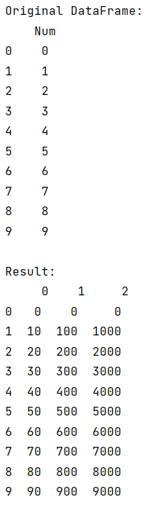# Pandas: Apply function that returns multiple values to rows in pandas DataFrame

Given a Pandas DataFrame, we have to apply function that returns multiple values to rows.
Submitted by Pranit Sharma, on July 17, 2022

Pandas is a special tool that allows us to perform complex manipulations of data effectively and efficiently. Inside pandas, we mostly deal with a dataset in the form of DataFrame. DataFrames are 2-dimensional data structures in pandas. DataFrames consist of rows, columns, and data.

## Problem statement

Given a Pandas DataFrame, we have to apply function that returns multiple values to rows.

## Solution approach

Whenever we want to perform some operation on the entire DataFrame, we either use apply method. It is used on the grouped objects in pandas DataFrame.

### The apply() method

The apply() method passes the columns of each group in the form of a DataFrame inside the function which is described in apply() method. The function which is described inside the apply() method returns a series or DataFrame (NumPy array or even a list).

## Apply function that returns multiple values to rows

To apply a function that returns multiple values to rows in pandas DataFrame, we will define a function for performing some operations on the values, and then finally we will return all the values in the form of a series.

Note

To work with pandas, we need to import pandas package first, below is the syntax:

```import pandas as pd
```

Let us understand with the help of an example,

## Python program to apply function that returns multiple values to rows in pandas DataFrame

```# Importing Pandas package
import pandas as pd

# Create a dictionary
d= {'Num': [ i for i in range(10)]}

# Create DataFrame
df = pd.DataFrame(d)

# Display DataFrame
print("Original DataFrame:\n",df,"\n")

# Defining a function
def function(n):
a=n*10
b=n*100
c=n*1000
return pd.Series([a,b,c])

# Using apply method
result = df['Num'].apply(function)

# Display result
print("Result:\n",result)
```

### Output